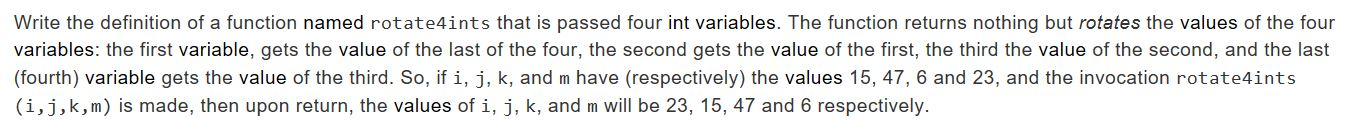# Write the definition of a function named rotate4ints that is passed four int variables. The function returns nothing but rotates the values of the fourvariables: the first variable, gets the value of the last of the four, the second gets the value of the first, the third the value of the second, and the last(fourth) variable gets the value of the third. So, if i, j, k, and m have (respectively) the values 15, 47, 6 and 23, and the invocation rotate4ints(i,j,k,m) is made, then upon return, the values of i, j, k, and m will be 23, 15, 47 and 6 respectively.

Question
18 views

Help.help_outlineImage TranscriptioncloseWrite the definition of a function named rotate4ints that is passed four int variables. The function returns nothing but rotates the values of the four variables: the first variable, gets the value of the last of the four, the second gets the value of the first, the third the value of the second, and the last (fourth) variable gets the value of the third. So, if i, j, k, and m have (respectively) the values 15, 47, 6 and 23, and the invocation rotate4ints (i,j,k,m) is made, then upon return, the values of i, j, k, and m will be 23, 15, 47 and 6 respectively. fullscreen
check_circle

Step 1

Below is the algorithm:-

start
function rotate4ints(i,j,k,m)
declare integer temp1=i
i=m
declare integer temp2=j
j=temp1
declare integer temp3=k
k=temp2
m=temp3
display "The values after rotation are: "+i+" "+j+" "+k+" "+m
end function
end

Step 2

Below is the java code for the above algorithim:-

public class Rotate{
public void rotate4ints(int i,int j,int k,int m){
int temp1=i; //copy i in temp1
i=m; //copy m in i
int temp2=j; //copy j in temp2
j=temp1; //copy temp1 in j
int temp3=k; //copy k in temp3
k=temp2; //copy temp2 in k
m=temp3; //copy temp3 in m
System.out.println("The values after rotation are: "+i+" "+j+" "+k+" "+m);
}
}

Step 3

Below is the screenshot...

### Want to see the full answer?

See Solution

#### Want to see this answer and more?

Solutions are written by subject experts who are available 24/7. Questions are typically answered within 1 hour.*

See Solution
*Response times may vary by subject and question.
Tagged in

### Programing Language